• 数据结构与算法实验指导 V2016 实验四 串 实验目的 1掌握串的存储表示及基本操作 2掌握串的两种模式匹配算法 BF 和 KMP 3了解串的应用 实验学时 2 学时 实验预习 回答以下问题 1串和子串的定义 串的定义串是由零个或...
• 数据基本结构设计计算机编程中加工处理对象是数据，而数据具有一定组织结构，所以学习编写程序仅仅了解计算机语言是不够，还必须掌握数据组织、储存和运算的一般方法。在掌握了这些基础方法前提下，才能灵活...
• ﻿﻿ 字符串 字符串（String），是由零个或多个字符组成的有限序列。它是编程语言中表示文本的数据类型。...通常以串的整体作为操作对象，如：在串中查找某个子串、求取一个子串、在...串的两种基本的存储方式是顺
﻿﻿

字符串

字符串（String），是由零个或多个字符组成的有限序列。它是编程语言中表示文本的数据类型。

通常以串的整体作为操作对象，如：在串中查找某个子串、求取一个子串、在串的某个位置上插入一个子串以及删除一个子串等。两个字符串相等的充要条件是：长度相等，并且各个对应位置上的字符都相等。设p、q是两个串，求q在p中首次出现的位置的运算叫做模式匹配。串的两种最基本的存储方式是顺序存储方式和链接存储方式。

形式理论

设Σ是叫做字母表的非空有限集合。Σ的元素叫做“符号”或“字符”。在Σ上的字符串（或字）是来自Σ的任何有限序列。例如，如果Σ = {0, 1}，则0101是在Σ之上的字符串。

字符串的长度是在字符串中字符的数目（序列的长度），它可以是任何非负整数。“空串”是在Σ上的唯一的长度为0的字符串，并被指示为ε或λ。

在Σ上的所有长度为n的字符串的集合指示为Σn。例如，如果Σ
= {0, 1}则Σ2 = {00, 01, 10, 11}。注意Σ0 = {ε}对于任何字母表Σ。

在Σ上的所有任何长度的字符串的集合是Σ的Kleene闭包并被指示为Σ*。依据Σn,
\Sigma^{*} = \bigcup_{n=0}^{\infty}\Sigma^{n}。例如，如果Σ = {0,1}则Σ*
= {ε, 0, 1, 00, 01, 10, 11, 000, 001, 010, 011,…}。尽管Σ*自身是可数无限的，Σ*的所有元素都有有限长度。

在Σ上一个字符串的集合（就是Σ*的任何子集）被称为在Σ上的形式语言。例如，如果Σ
= {0, 1}，则带有偶数个零的字符串的集合（{ε, 1, 00, 11, 001, 010, 100,
111, 0000, 0011, 0101, 0110, 1001, 1010,1100, 1111,…}）是在Σ上的形式语言。

串接和子串

“串接”是Σ*上的重要二元运算。对于Σ*中的两个字符串s和t，它们的串接被定义为在s中的字符序列之后跟随着t中的字符序列，并被指示为st。例如，Σ
= {a, b,…, z}，并且s = bear且t
= hug，则st = bearhug而ts = hugbear。

字符串串接是结合性的，但非交换性运算。空串充当单位元；对于任何字符串s，有εs
= sε = s。所以，集合Σ*和串接运算形成了幺半群，就是从Σ生成的自由幺半群。此外，长度函数定义从Σ*到非负整数的幺半群同态。

字符串s被称为是字符串t的“子串”或“因子”，如果存在（可能为空）字符串u和v使得t
= usv。“是其子串”关系定义了在Σ*上的偏序，其最小元是空串。

词典排序

经常需要定义在字符串集合上的次序。如果字符表Σ有一个全序（cf.
字母序），则可以定义在Σ*上的叫做词典序的全序。注意因为Σ是有限的，总是可以定义在Σ继而在Σ*上的良好次序。例如，如果Σ
= {0, 1}并且0 < 1，则Σ*的词典次序是ε
< 0 < 00 < 000 <…< 011 < 0110<…< 01111 <…<
1 < 10 < 100 <…< 101 <…< 111…

字符串数据类型[编辑]

字符串数据类型是建模在形式字符串的想法上的数据类型（Data type）。字符串是几乎在所有编程语言中可以实现的非常重要和有用的数据类型。在某些语言中它们可作为基本类型获得，在另一些语言中做为复合类型获得。多数高级语言的语法允许通常用某种方式引用起来的字符串来表示字符串数据类型的实例；这种元字符串叫做“文字”或“字符串文字”。

字符串长度

尽管形式字符串可以有任意（但有限）的长度，实际语言的字符串的长度经常被限制到一个人工极大值。一般的说，有两种类型的字符串数据类型：“定长字符串”，它有固定的极大长度并且不管是否达到了这个极大值都使用同样数量的内存；和“变长字符串”，它的长度不是专断固定的并且依赖于实际的大小使用可变数量的内存。在现代编程语言中的多数字符串是变长字符串。尽管叫这个名字，所有变长字符串还是在长度上有个极限，一般的说这个极限只依赖于可获得的内存的数量。

字符编码

历史上，字符串数据类型为每个字符分配一个字节，尽管精确的字符集随着区域而改变，字符编码足够类似得程序员可以忽略它—同一个系统在不同的区域中使用的字符集组要么让一个字符在同样位置，要么根本就没有它。这些字符集典型的基于ASCII码或EBCDIC码。

意音文字的语言比如汉语、日语和朝鲜语（合称为CJK）的合理表示需要多于256个字符（每字符一个字节编码的极限）。常规的解决涉及：保持对ASCII码的单字节表示，并使用双字节来表示CJK字形。现存代码在用到它们会导致一些字符串匹配和切断上的问题，严重程度依赖于字符编码是如何设计的。

1.某些编码比如EUC家族保证在ASCII码范围内的字节值只表示ASCII字符，使得使用这些字符作为字段分隔符的系统得到编码安全。其他编码如ISO-2022和Shift-JIS不做这种担保，使得基于字节的代码做的匹配不安全。

2.另一个问题是如果一个字符串的开头被删除了，对解码器的重要指示或关于在多字节序列中的位置的信息可能就丢失了。

3.另一个问题是如果字符串被连接到一起（特别是在被不知道这个编码的代码截断了它们的结尾之后），第一个字符串可能不能导致编码器进入适合处理第二个字符串的状态中。

Unicode也有些复杂的问题。多数语言有Unicode字符串数据类型（通常是UTF-16，因为它在Unicode补充位面介入之前就被增加了）。在Unicode和本地编码之间转换要求理解本地编码，这对于现存系统要一起传输各种编码的字符串而又没有实际标记出它们用了什么编码就是个问题。

实现

某些语言如C++把字符串实现为可以用于任何基本类型的模版，但这是个例外而不是规则。

如果一个面向对象语言把字符串表示为对象，它们叫做“可变的”（mutable），如果值可以在进行时间变更，叫做“不变的”（immutable），如果这个值在建立后就冻结了。例如，Ruby有可变字符串，而Python的字符串是不可变的。

其他语言，最著名的有Prolog和Erlang，避免实现字符串数据类型，转而采用把字符串表示为字符代码的列表的约定。

表示法

一种常用的表示法是使用一个字符代码的数组，每个字符占用一个字节（如在ASCII代码中）或两个字节（如在unicode中）。它的长度可以使用一个结束符（一般是NUL，ASCII代码是0，在C编程语言中使用这种方法）。或者在前面加入一个整数值来表示它的长度（在Pascal语言中使用这种方法）。

这是一个用NUL结束的字符串的例子，它用10个byte存储，用ASCII表示法：

F R A N K NUL k e f w

46 52 41 4E 4B 00 6B 66 66 77

上面的字符串的长度为5个字符，但注意它占用6个字节。结束符后的字符没有任何意义。

这是相同的Pascal字符串：

length F R A N K k e f w

05 46 52 41 4E 4B 6B 66 66 77

当然，可能还有其它的表示法。使用树和列表可以使得一些字符串操作（如插入和删除）更高效。


展开全文• 数据结构与算法实验指导V2016 PAGE 10 实验四 串 实验目的 1掌握串的存储表示及基本操作 2掌握串的两种模式匹配算法BF和KMP 3了解串的应用 实验学时 2学时 实验预习 回答以下问题 1串和子串的定义 串的定义串是由零...
• 那么就有字符串数据的加减乘除运算基本结构BigData.h有两种成员变量; long long _value; string _strData;两种构造函数：字符串，int 构造函数已经处理过字符串，正数的字符串是，”+…….”,负数
在日常计算中，一般来说，数据不会太大，即使再大long long 8个字节也够用，但是现在来说数据很大，一般的数据类型都表示不了，那么就用字符串来表示数据。

那么就有字符串数据的加减乘除运算。

基本结构BigData.h

有两种成员变量;
long long  _value;
string  _strData;

两种构造函数：字符串，int 构造函数已经处理过字符串，正数的字符串是，”+…….”,负数的字符串是”-……….”。

#pragma once
#define _CRT_SECURE_NO_WARNINGS 1
#include<iostream>
using namespace std;

typedef long long INT64;

class BigData
{
public:

BigData(INT64 data);
BigData(const string& str);
BigData operator+(const BigData& b);
BigData operator-(const BigData& b);
BigData operator*(const BigData& b);
BigData operator/(const BigData& b);
BigData& operator=(const BigData& b);
friend ostream& operator<<(ostream& out, const BigData& bigdata);

private:
//判断是否越界
bool IsINT64Overflow()const;

//Add  这些函数的参数 肯定都带符号，
string Add(string left, string right);

string Sub(string left, string right);

string Mul(string left, string right);

string Div(string left, string right);

//判断被除数大于除数
bool isLeftBig(char* left, size_t Lsize, char* right, size_t Rsize);//left前面不为0

//计算商值，并且返回余数
char loopsub(char*& left, size_t& Lsize, char*& right, size_t Rsize);

private:
INT64 _value;
string _strData;
};

构造函数以及输出运算符重载

INT64 UN_INT = 0xcccccccccccccccc;
const INT64 MaxValue = 9223372036854775807;
const INT64 MinValue = -9223372036854775807;

ostream& operator<<(ostream& out, const BigData& bigdata)
{
char* pData = (char*)bigdata._strData.c_str();
if (*pData == '+')
pData++;
out << pData;
return out;
}

BigData::BigData(INT64 data = UN_INT)
:_value(data)
{
//数字放入字符串中
_strData = "+";
INT64 temp = _value;
if (temp < 0)
{
_strData = '-';
temp = 0 - temp;
}
else if (temp == 0)
_strData = "+0";
while (temp != 0)
{
_strData += temp % 10 + '0';
temp /= 10;
}
reverse(_strData.begin() + 1, _strData.end());
}

//模拟atoi
BigData::BigData(const string& strData)
: _value(0)
, _strData("+0")
{
// "123456789" "+123456890"  "12345asdfg123456"
// "000001234567"  "      12345678"
// "aa12345689" "000000000000000000"  "             "
if (strData.empty())
return;

char* pData = (char*)strData.c_str();
char symbol = *pData;
if (*pData == '-' || *pData == '+')
pData++;

//处理前置空格
while (isspace(*pData))
pData++;
if ('\0' == *pData)
return;

//处理 前置0
while ('0' == *pData)
pData++;
if ('\0' == *pData)
return;
//处理前置非数字
if (*pData > '9' || *pData < '0')
return;

//处理剩余部分
size_t Length = strlen(pData);
_strData.resize(Length + 1);//多加一个符号位
if (symbol == '-')
_strData = '-';
size_t count = 1;
while (*pData >= '0' && *pData <= '9')
{
_value = _value * 10 + *pData - '0';
_strData[count++] = *pData++;
}
_strData.resize(count);

if (symbol == '-')
_value = 0 - _value;
}

//判断是否越界
bool BigData::IsINT64Overflow()const
{
std::string strTemp("+9223372036854775807");
if (_strData == '-')
strTemp = "-9223372036854775808";

if (_strData.size() < strTemp.size())
return false;
else if (_strData.size() == strTemp.size() && _strData < strTemp)
return false;

return true;
}

BigData& BigData::operator=(const BigData& b)
{
if (this != &b)
{
BigData temp(b);
std::swap(_value, temp._value);
std::swap(_strData, temp._strData);
}
return *this;
}

加法
只有当符号位相同时，才进行加法。

字符串相加，比较容易实现，
(1)结果的字符串的位数要最大的那位，要多加一位，防止最后一位进位。
(1) 将字符串 位数大的 放在外面，
(2)设置一个进位累加器，相加。

string Add(string left, string right)
{
size_t Lsize = left.size();
size_t Rsize = right.size();
string res;
char symbol = 0;
//正常调用add的为同号，
//当为-时候，异号调用Add,位数相同时，符号位为left,位数不同时，符号位交换之前的left
if (Lsize < Rsize) //使left的位数大
{
if (left != right) //异号
symbol = left;
std::swap(Lsize, Rsize);
std::swap(left, right);
}
res.resize(Lsize + 1); //可能最后产生进位
if (symbol != 0)
res = symbol;
else
res = left;
char takeover = 0;//进位
for (size_t idx = 1; idx < Lsize; ++idx)
{
char temp = left[Lsize - idx] - '0' + takeover;
if (Rsize > idx)
temp += right[Rsize - idx] - '0';
takeover = temp / 10;
res[Lsize + 1 - idx] = temp % 10 + '0';
}
res = takeover + '0';
return res;
}
BigData BigData::operator+(const BigData& b)
{
if (!IsINT64Overflow() && !b.IsINT64Overflow())
{
//没有超出范围
if (_strData != b._strData)//异号
return BigData(_value + b._value);
else
{
//10 - 2 = 8, 7
//-10 - -2 = -8,-7
//同号 但是没有超出范围
if ((_strData == '+' && MaxValue - _value >= b._value) ||
(_strData == '-' && MinValue - _value <= b._value))
return BigData(_value + b._value);
}
}
if (_strData == b._strData) //同号
return BigData(Add(_strData, b._strData));
return BigData(Sub(_strData, b._strData));
}

减法
(1) 位数大的放在外面，
(2)在减法过程中，注意借位问题。

string Sub(string left, string right)
{
size_t Lsize = left.size();
size_t Rsize = right.size();
char symbol  = 0;
if (Lsize < Rsize || (Lsize == Rsize && strcmp(left.c_str() + 1, right.c_str() + 1) < 0))
{
std::swap(Lsize, Rsize);
std::swap(left, right);
if (left == right && left == '+')//小的减去大的，且同号的话，比如left = 5，right = 10，
symbol = '-';

if (left == right && left == '-')//比如left = -5，right = -10，
symbol = '+';
}
string res;
res.resize(Lsize);// 减去不可能产生进位
if (symbol != 0)
res = symbol;
else
res = left;

for (size_t idx = 1; idx < Lsize; ++idx)
{
char temp = left[Lsize - idx] - '0';
if (Rsize > idx)
temp -= right[Rsize - idx] - '0';
if (temp < 0)
{
int step = 1;//向前step位借位
while ((Lsize >(idx + step)) && left[Lsize - idx - step] == 0)
{
left[Lsize - idx - step] = '9';
step++;
}
left[Lsize - idx - step]--; //不为0时 要-1
temp += 10;
}
res[Lsize - idx] = temp % 10 + '0';
}
return res;
}
BigData BigData::operator-(const BigData& b)
{
if (!IsINT64Overflow() && !b.IsINT64Overflow())
{
//没有超出范围
if (_strData == b._strData)//同号号
return BigData(_value - b._value);
else
{
// [10,-10]  2和 -7
// -10 +2 = -8
// 10 + -7 = 3
//异号 但是没有超出范围
if ((_strData == '+' && MinValue + _value <= b._value) ||
(_strData == '-' && MaxValue + _value >= b._value))
return BigData(_value - b._value);
}
}
if (_strData == b._strData) //同号
return BigData(Sub(_strData, b._strData));
return BigData(Add(_strData, b._strData));
}

乘法
(1) 同样大的放在外层left
(2)内层的每个位于外层left相乘，然后与res中相加，

string Mul(string left, string right)
{
size_t LSize = left.size();
size_t RSize = right.size();
if (LSize > RSize) //位数小的放到外层循环
{
std::swap(LSize, RSize);
std::swap(left, right);
}
char takeover = 0; //进位

size_t resSize = LSize + RSize - 1;
string res(resSize, '0');//全都初始化'0';
size_t offset = 0;//结果移位
for (size_t i = 1; i < LSize; ++i)
{
char cleft = left[LSize - i] - '0';// 外层循各个位的值
takeover = 0;
for (size_t j = 1; j < RSize; ++j)
{
char resBit = res[resSize - offset - j] - '0';
char mulBit = (right[RSize - j] - '0') * cleft + takeover + resBit;
takeover = mulBit / 10;
res[resSize - offset - j] = mulBit % 10 + '0';
}
offset++;
}
//最后一次进位没有写入到结果中
res = takeover + '0';
//符号
if (left == right)
res = '+';
else
res = '-';
return res;
}
BigData BigData::operator*(const BigData& b)
{
if (_value == 0 || b._value == 0)
return BigData(0);
else if (strcmp(_strData.c_str() + 1, "1") == 0)
return BigData(b._strData);
else if (strcmp(b._strData.c_str() + 1, "1") == 0)
return BigData(_strData);
return BigData(Mul(_strData, b._strData));
}

除法
借助两个函数，
isLeftBig：判断左边的数据是否大于右边的数据
loopsub：循环减去右边的值，计算差值。直到需要借位。

    //判断被除数大于除数
bool isLeftBig(char* left, size_t Lsize, char* right, size_t Rsize)//left前面不为0
{
if (Lsize == Rsize && strncmp(left, right, Rsize) >= 0)
return true;
else if (Lsize > Rsize)
return true;

return false;
}
//计算商值，并且返回余数
char loopsub(char*& left, size_t& Lsize, char*& right, size_t Rsize)
{
char count = '0';//相当于商值
while (isLeftBig(left, Lsize, right, Rsize))
{
for (size_t i = 0; i < Lsize; ++i)
{
char temp = left[Lsize - 1 - i] - '0';
if (i < Rsize)
temp -= (right[Rsize - 1 - i] - '0');
if (temp < 0)
{
//向前借位
size_t step = 1;//借的步数
while ((1 + i + step < Lsize) && left[Lsize - 1 - i - step] == 0) //肯定可以借到，因为左边大于右边
{
left[Lsize - 1 - i - step] = '9';
step++;
}
left[Lsize - 1 - i - step]--;
temp += 10;
}
left[Lsize - 1 - i] = temp + '0';
}
count++;
while (Lsize > 0 && *left == '0') //去除前面的0
{
left++;
Lsize--;
}
if (Lsize == 0)
break;
}
return count;

}
string Div(string left, string right)
{
char symbol = '+';
if (left != right)
symbol = '-';
string res;
res.append(1, symbol);
char* pleft = (char*)left.c_str() + 1;
char* pright = (char*)right.c_str() + 1;
size_t Lsize = left.size() - 1;
size_t Rsize = right.size() - 1;
size_t len = Rsize;

while (*(pleft + len - 1) != '\0') //感觉这可能越界
{
if (!isLeftBig(pleft, len, pright, Rsize))
{
if (*pleft == '0')
pleft++;
else
len++;
res.append(1, '0');
continue;
}
else
{
res.append(1, loopsub(pleft, len, pright, Rsize));
len++;
}
}
return res;
}
BigData BigData::operator/(const BigData& b)
{
//商值为0,1，-1
char* left = (char *)_strData.c_str();
char* right = (char *)b._strData.c_str();
//除数不能为0
if (b._value == 0)
{
cout << "除数不能为0" << endl;
return BigData(0);
}
//商值为0
if (_strData.size() < b._strData.size())
return BigData(0);
else if (_strData.size() == b._strData.size() && strcmp(_strData.c_str() + 1, b._strData.c_str() + 1) < 0)
return BigData(0);
//商值为 1
if (strcmp(left, right) == 0)
return BigData(1);
//商值为-1
if (strcmp(_strData.c_str() + 1, b._strData.c_str() + 1) == 0 && *left != *right)
return BigData(-1);
else if (b._value == 1) //被除数为1
return BigData(_strData);
else if (b._value == -1) //被除数为-1
return BigData(b._strData);

return BigData(Div(_strData, b._strData));
}
展开全文• 通过训练，加深理解并把握串的基本运算的特点。 二、实验内容 【问题描述】 本题目中的串编辑要求对串实现以下两种功能： 本题目中的串编辑要求对串实现以下两种功能： ⑴插入：把一个字符串插入到给定串的指定位置...
一、实验目的
通过训练，加深理解并把握串的基本运算的特点。
二、实验内容
【问题描述】
本题目中的串编辑要求对串实现以下两种功能：
本题目中的串编辑要求对串实现以下两种功能：
⑴插入：把一个字符串插入到给定串的指定位置；
⑵删除：将串中某指定位置开始的若干字符从串中删除；
【参考程序】
#include
#include <conio.h>
#include <string.h>
using namespace std;
class String
{
public:
String(){};
String(const String&s);
~String(){};
String SubString(int pos,int num);
void Insert(String t,int pos);
void Delete(int pos,int num);
void Creat();
void Display();
void Replace(String t, int pos);
private:
char *str;
int size;
};
String::String(const String&s)
{
size=s.size;
str=new char[size+1];
strcpy(str,s.str);
}
//生成新字符串函数
void String::Creat()
{
//char s=;//不能只想一字符串常量
chars=new char;
cin>>s;
size=strlen(s);
str=new char[size+1];//还包括’\0’的空间
if (str==0)
{
cout<<“没有申请到空间！”;
}
strcpy(str,s);
delete[] s;
}
//输出
void String::Display()
{
int i;
for (i=0;i<size;i++)
{
cout<<str[i];
}
cout<<endl;
}
//求子串
String String::SubString(int pos,int num)
{
String cs;
if (pos>=0&&pos<size)
{
cs.str=new char[num+1];
for (int i=0;i<num;i++)
{
cs.str[i]=str[pos+i];
}
cs.str[num]=’\0’;
cs.size=num;
return cs;
}
else
{
cs.size=-1;
return cs;
}
}
//插入运算：在串对象s的pos位置后插入一个串t
void String::Insert(String t,int pos)
{
if (pos<0||pos>size)
{
cout<<“输入的位置非法”;
return;
}
String cs;
cs.size=size+t.size;
cs.str=new char[cs.size+1];
for(int i=0;i<=pos-1;i++)
{
cs.str[i]=str[i];
}
for(int j=0;j<t.size;j++)
{
cs.str[j+pos]=t.str[j];
}
for(int k=pos;k<size;k++)
{
cs.str[k+t.size]=str[k];
}
    str=cs.str;
size=cs.size;

}
//删除：删除串中的一个串
void String::Delete(int pos,int num)
{
if (pos<0||pos>size)
{
cout<<“输入的位置非法”;
return;
}
else
{
String cs;
cs.size=size-num;
cs.str=new char[cs.size+1];
for(int i=0;i<=pos-1;i++)
{
cs.str[i]=str[i];
}
for(int k=pos;k<=size-num;k++)
{
cs.str[k]=str[k+num];
}
            str=cs.str;
size=cs.size;
}

}
//置换指定位置的若干字符串
void String::Replace(String t, int pos)
{
int i, j;
if (pos > 0)
{
if (this->size >= pos + t.size)
{
for (i = 0; i < this->size; i++)
{
if (i >= pos - 1)
{
for (j = 0; j < t.size; j++)
{
this->str[i++] = t.str[j];
}
break;
}
}
}
else
{
this->size = pos + t.size;
for (i = 0; i < this->size; i++)
{
if (i >= pos - 1)
{
for (j = 0; j < t.size; j++)
{
this->str[i++] = t.str[j];
}
this->str[i] = ‘\0’;
break;
}
}
}
}
else
{
cout << "置换失败!\n";
}

}
//主函数
int main(int argc, char* argv[])
{
int pos, num, k;
String s, s1, t;
do {
cout << “\n\n    1.生成字符串”;
cout << “\n\n    2.取子串”;
cout << “\n\n    3.插入子串s1”;
cout << “\n\n    4.删除子串”;
cout << “\n\n    5.置换子串”;
cout << “\n\n    6.结束程序”;
cout << "\n******************************** ";
cout << “\n    请输入你的选择(1,2,3,4,5,6)”;  cin >> k;
switch (k) {
case 1: {cout << “请输入一个字符串：”;
s.Creat();
cout << "字符串为：       ";     s.Display();
}break;
case 2: { cout << “请输入子串的截取位置pos及子串长度num” << endl;
cin >> pos >> num;
t = s.SubString(pos, num);
cout << "你所取的子串为：　　";  t.Display();
}break;
case 3: { cout << “请输入子串插入位置pos” << endl;
cin >> pos;
cout << "请输入要插入的子串:  ";   s1.Creat();
s.Insert(s1, pos);
cout << "插入后的字符串为：   ";  s.Display();
}break;
case 4: { cout << “请输入要删除子串的开始位置pos及子串长度num” << endl;
cin >> pos >> num;
s.Delete(pos, num);
cout << "删除后的字符串为：   ";
s.Display();
}break;
case 5: { cout << “请输入子串置换位置pos” << endl;
cin >> pos;
cout << "请输入要置换的子串:  ";   s1.Creat();
s.Replace(s1, pos);
cout << "置换后的字符串为：   ";  s.Display();
}break;
default:break;
} //switch
cout << "\n--------------------------------- ";
} while (k >= 1 && k < 6);
cout << “\n          再见!”;
cout << “\n     按任意键，返回。”;
return 0;
}


展开全文• Python基础学习记录（4/17）——字符串...在所有编程语言中，字符串都是最基本的数据结构之一。 类似与元组具有不可变性，每个字符一旦创建，不可通过索引对其做任何修改 创建字符串可使用成对单引号或双引号，...
Python基础学习记录（4/17）——字符串与列表字符串python中常用的转义字符python字符串运算字符串方法
字符串
字符串（String)是序列的一种,实际上字符串是单个字符的序列，可以简单理解为它是由多个单个字符合并而来。在所有编程语言中，字符串都是最基本的数据结构之一。

类似与元组具有不可变性，每个字符一旦创建，不可通过索引对其做任何修改
创建字符串可使用成对的单引号或双引号，两种方法相同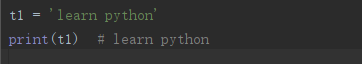当字符串中有双引号时，可用单引号避免歧义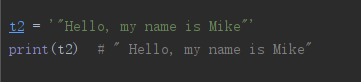如果字符串中出现单引号或者双引号，可以用转义符\对字符串中的字符进行转义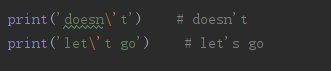python中常用的转义字符

转义字符
描述

\\
反斜杠符

\’
单引号

\"
双引号

\n
换行符

\t
横向制表符

\r
回车，特殊字符失效

\f
换页

\b
退格

python字符串支持跨行，常用的方法使用’’’…’’'或者"""…"""，字符串中可以包含换行符、制表符以及其他特殊字符

字符串可以通过[ ]来访问其中的有序数据，支持索引和切片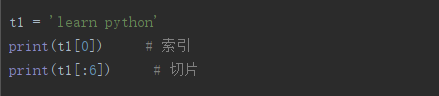python字符串运算

操作符
描述

+
字符串拼接

*
重复输入字符串

%
格式化字符串，%放置在字符串左侧简单格式化字符串，而右侧则放置希望格式化的值

[]
索引

[:]
切片：遵循左闭右开原则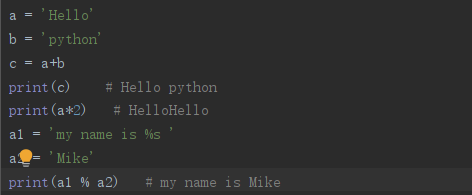字符串方法

函数名称
函数说明

S.fand(sub,[,start[,end]])
返回在字符串中找到的子字符串sub的最低索引，使得sub包含在切片是s[start:end]中，如果未找到sub，则返回-1

S.split([sept[,maxsplit]])
返回字符串中的单词列表，使用seq作为分隔符字符串。如果给出maxsplit，则至多拆分maxsplit次（因此列表中将最多有maxsplit+1个元素）。如果没有指定maxsplit或为-1，那么分隔符的数量没有限制

S.join(iterator)
连接字符串数组。将字符串、元组、列表中的元素以指定的字符（分隔符）连接成一个新的字符串

S.strip([chars])
返回字符串的一个副本，删除前导和尾随字符。chars参数是一个字符串，指定要移除的字符集。如果省略或为None，则chars参数默认为删除空白符

S.lower()
将字符串中所有大写字符变为小写

S.isalnum
如果字符串中至少有一个字符，并且所有字符都是数字或者字母，则返回ture，否则返回false

S.count(sub[,start[,end]])
发明会在[start,end]范围内的子串sub非重叠出现的次数。可选参数start和end都以切片表示法解释

S.replace(old,new[,count])
返回字符串的一个拷贝，其中所有的子串old通过new替换。如果指定了可选参数count，则只有前面的count个出现被替换


展开全文• Python有五种数据结构，分别是数字、字符串、列表、元组、字典。 1、数字 Python Number 数据类型用于存储数值。数据类型包括长整型、整型、浮点型、复数。 主要有块要涉及到数字运算： 数学相关函数Python ...python
• 5、 能行性(realizability)：算法中每一步都可以通过已经实现的基本运算的有 限次运行来实现。 算法含义与程序非常相似，但二者有区别。一个程序不一定满足有穷性。 例如操作系统，只要整个系统不遭破坏，它将...
• 我所提到的“over”、“end”、“lie”其实就是“lover”、“friend”、“believe”这些单词字符串的子串。 5.3串的比较 126 5.4串的抽象数据类型 127 5.5串的存储结构 128 感情上发生了问题，为了向女友解释...
• 实现一个基本的计算器来计算一个简单的字符串表达式值。 示例 : 输入: “6+5/3-2*2” 输出: 3 因为乘除优先级大于加减,我们现实现加减运算 我们使用数据结构栈Stack,是先进后出,有进栈和出栈两种 方法一般用到...java
• 熟练掌握在两种存储结构上实现栈和队列的基本运算；学会利用栈和队列解决一些实际问题。 运算实现 时数 2 性质 验证 内容：1、若X和Y是用结点大小为1单链表表示的串，设计算法找出X中第一个不在Y中出现的字符...
• 本系统对屏幕设计的基本原则是集数据结构、算法和其他重要信息（如栈等）于同一屏幕。一般情况下演示屏由图示、算法和变量三个窗口组成，特殊情况下将根据演示内容适当增加。一般情况下， 左侧图示窗口显示演示数据...
• 简单值（原始值）：包含字符串、数字和布尔值，此外，还有个特殊值——null（空值）和 undefined（为定义）。 复杂的数据结构（泛指对象）：包括狭义对象、数组和函数。 基本类型 JavaScript定义了 6 种基本...javascript js java
• 熟练掌握在两种存储结构上实现栈和队列的基本运算；学会利用栈和队列解决一些实际问题。 五：内容：1、若X和Y是用结点大小为1单链表表示的串，设计算法找出X中第一个不在Y中出现的字符。 2、设计一算法，在顺序...
• 33. 字符串的长度是指（ ）。 (A) 串中不同字符的个数 (B) 串中不同字母的个数 (C) 串中所含字符的个数 (D) 串中不同数字的个数 34. 队列是一（ ）的线性表。 (A) 先进先出 (B) 先进后出 (C) 只能插入 (D) 只能...
• 本系统对屏幕设计的基本原则是集数据结构、算法和其他重要信息（如栈等）于同一屏幕。一般情况下演示屏由图示、算法和变量三个窗口组成，特殊情况下将根据演示内容适当增加。一般情况下， 左侧图示窗口显示演示数据...
• 6．数据结构中评价算法的两个重要指标是（时间复杂度和空间复杂度） 【北京理工大学 2001 七、1（2分）】 7. 数据结构是研讨数据的_（1）物理结构_和_（2）逻辑结构 _，以及它们之间的相互关系，并对与这种结构定义...
• 下面先实现最基本的加减乘除（重点分析如何实现字符串读入中多位数处理方式）由于实现方法有三，一个是利用个栈实现代码，一个是中缀转后缀，一个是顺序栈实现。本文先用个栈实现 在这里插入代码片 #...
• 第1章 数据结构. 1 1.1 数组和字符串 2 1.1.1 一维数组倒置 2 范例1-1 一维数组倒置 2 ∷相关函数：fun函数 1.1.2 一维数组应用 3 范例1-2 一维数组应用 3 1.1.3 一维数组高级应用 5 范例1-3 一维数组...
• <br>实验二 单链表结构及计算 实验目的: 通过实验掌握下列知识: 1、熟悉线性表的基本运算两种存储结构（顺序结构和链式结构）上实现； 2、继续熟悉VC编程、编译和调试环境； 内容及步骤:...
• 3.4 两种实现对比.....................................................................................................53 3.4.1 基于时间比较.............................................................
• 019.MFC_两种对话框.mp4 020.MFC_文件对话框.mp4 021.MFC_字体和颜色对话框.mp4 022.MFC_图片控件_动画控件.mp4 023.MFC_属性页控件.mp4 024.讲MFC_窗口指针.mp4 025.MFC_窗口操作.mp4 026.MFC_发送消息.mp4...
• 目录 第一章 Java与面向对象程序... 3.3.3 线性表单链表实现 两种实现对比 3.4.1 基于时间比较 3.4.2 基于空间比较 链接表.54 3.5.1 基于结点操作 3.5.2 链接表接口 3.5.3 基于双向链表实现链接表...

# 数据结构字符串的两种基本运算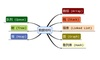数据结构 订阅